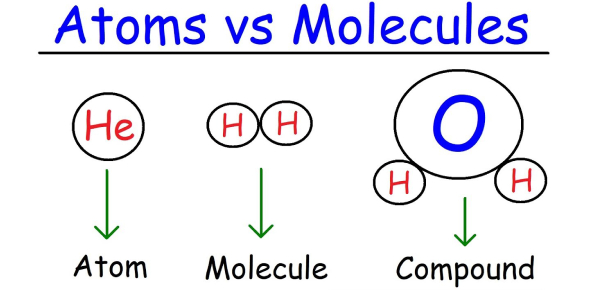# MCQ On Elements And Atomic Structure! Trivia Quiz

10 Questions | Attempts: 485SettingsHow good are you with the chemical elements, their atomic structure, and atomic number? Would you be able to pass this quiz? Regarding this quiz, you should know what an atomic number is, which element is number 12, what is discovered in the nucleus, the volume and mass of an atom, and what is isotopes. This outstanding quiz is all about elements and atomic structure. All the best.

• 1.
Atomic number is ______________
• A.

Proton number

• B.

Mass number

• C.

Subscript

• D.

Electron number

• E.

None of the above

• 2.
You learn that an element has a mass number of 12. What element is it?
• A.

Hydrogen

• B.

Magnesium

• C.

Carbon

• D.

Oxygen

• E.

Cannot be determined

• 3.
All of the following are found in the nucleus EXCEPT ______.
• A.

Protons

• B.

Neutrons

• C.

Electrons

• D.

Attomic number

• E.

A and B

• 4.
The volume of an atom consists mostly of ______, and the mass of an atom is accounted for mostly by ______.
• A.

Protons and neutrons; empty space

• B.

Empty space; protons and neutrons

• C.

Protons and neutrons; electrons

• D.

Empty space; electrons

• E.

None of the above

• 5.
The atomic mass of an element is a whole number.
• A.

True

• B.

False

• 6.
Isotopes have the same atomic numbers, but different mass numbers.
• A.

True

• B.

False

• 7.
The nucleus of an atom takes up most of its volume and accounts for most of its mass.
• A.

True

• B.

False

• 8.
Isotopes are represented by the mass number written as a superscript and atomic number as a subscript written next to the element's chemical symbol.
• A.

True

• B.

False

• 9.
The number of neutrons in an atom can be calculated by subtracting the atomic number from the mass number.
• A.

True

• B.

False

• 10.
Isotopes have the same number of protons and neutrons but differ in their number of electrons.
• A.

True

• B.

False

## Related TopicsBack to top
×

Wait!
Here's an interesting quiz for you.